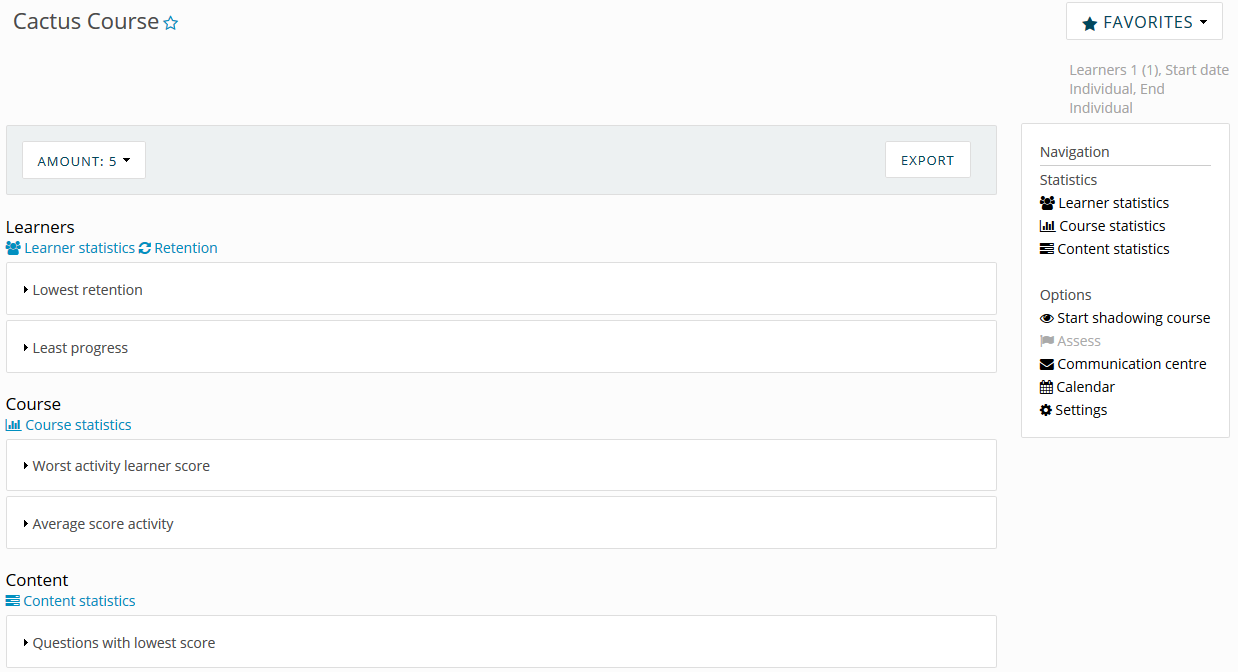You have access to several statistics that allow you to gain a better insight in the progress of the learners. Go to the tab Instruct and then go to the Dashboard of the course where you want to check the statistics.

### Statistics dashboard

Here you have an overview in which you can find the statistics. You can export statistics by clicking on Export. The overview consists of three subjects: Learners, Course and Content. At the right side of the overview you find another overview with all the options. The functionalities of the subjects are listed below.### Learners

If you click on Retention you can check the retention on learning objectives for all the learners in one overview. You can export it as a CSV file.
Learner statistics: check the progress and results of all learners. In the right column you see the general progress of all learners. In the left column you can check the progress and results per learner. You can check the General progress, Activities and the Memotrainer statistics there.

The numbers you see in the Summary give you a clear overview of the progress of the learner.

• Activities: The general progress on the activities available for the learner.
• Retention: Gives the percentage of all questions that has been answered on the indicated training level. The knowledge intake is being built up using the MemoTrainer. Also read the article 'What is the MemoTrainer?'
• Efficiency: Is built up from the number of times a question has been answered correctly, divided by the number of attempts.At the bottom of the overview per learner, you will find some graphs that give you a visual insight in the results of the last MemoTrainings by the learner.#### Objectives

When on the tab Objectives you can see the progress on the learning objectives, if they are being used within the course. Where the light, striped bar stops indicates when enough retention is accumulated to reach the goal. The green colored, striped part indicates the amount of retention accumulated so far by the learner for the particular goal.

## Course

Check which activities are scored on the best or the worst. In the overview on the left side, the course blocks with the according activities are shown. Check here how many questions are answered correctly or incorrectly. You can check it per learner or per question.

You can also check the p-value. The p-value is a number between 0 and 1 that indicates the difficulty level of the question. The lower the p-value, the more difficult the learners find the question. The p-value is calculated by dividing the number of times a question has been answered correctly by the number of times the question has been answered. An example: when a question has been answered 20 times, 12 of which correctly, the p-value is 0.6 (12/20=0.6).### Content

Check which questions are considered difficult or easy by the learners. You see the name of the questions, ID, p-value (see below), correctly answered, incorrectly answers and total amount of answers.The p-value is a number between 0 and 1 that indicates the difficulty level of the question. The lower the p-value, the more difficult the learners find the question. The p-value is calculated by dividing the number of times a question has been answered correctly by the number of times the question has been answered. An example: when a question has been answered 20 times, 12 of which correctly, the p-value is 0.6 (12/20=0.6).